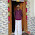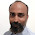## Sunday, 21 July 2013

### The on-board IR LED

We have an on-board IR LED on the Induino R3 Board. When we were designing the first Induino Board, we thought it would be fun to be able to control a television from the board and ended up adding the IR LED to the board. On top of being able to control devices with remote control, the IR LED can be combined with the TSOP to build a simple wireless communication system. However to try the wireless communication system, you would require two Induino R3 Boards.

The IR LED is connected to digital pin 14 (A0).  A Few things to note about the IR LED
• It is not a visible spectrum LED, so you will not be able to see it glowing with your naked eyes, if you look at the IR LED through a camera, you will be able to spot a Pink glow - that's the IR LED glowing.
• The IR LED on the Induino R3 board is driven by a simple driver circuitry and might not be as powerful as the one in your remote. You might have to point it in a straight line to get it working.
Understanding Frequency
The Sony SIRC protocol works at 40Khz...most remote controls work at their specific frequencies. TV remotes in India are generally between 32- 40Khz frequecny.

So whats this frequency all about?
40KHz means 40,000 Cycles in 1 second. Each Cycle represents a ON signal followed by a OFF signal. The signals could be of equal duration.

Here's another image of the Sony signal train

If you look at the image, you can see the the 1.2ms high of the Logical '1' has further black lines with spaces in between. These correspond to the ON/OFF cycles. The space between these is what is called the frequency. The frequency of occurrence of a ON/OFF cycle is what it means.

So How do we generate it?
Lets do some calculation,
40,000 Cycles in 1 Seconds => 1000 milliseconds  => 1000 x 1000 microseconds
so each cycle if for a duration of 25 microseconds.

We can produce this frequency if we can make a pin go high for 13 microseconds followed by low for 12 microseconds.

If we can do this for 2.4 milliseconds then we can generate a Start bit, if we can do this for 1.2 milliseconds then we can generate a Logical '1', and for 0.6 milliseconds, we can generate a Logical '0'.

Now that we know that each cycle will take 25 microseconds lets calculate the number of cycles required for generating the 2.4 milliseconds start bit

2.4 milliseconds / 25microseconds => 2400 microseconds / 25 microseconds => 96 cycles
1.2 milliseconds / 25microseconds => 1200 microseconds / 25 microseconds => 48 cycles
0.6 milliseconds / 25microseconds => 600 microseconds / 25 microseconds => 24 cycles

If you noticed, we are working with microseconds, we would need a new function to work with these

delaymicroSeconds(time in microseconds) => the delayMicroseconds() function takes a parameter and produces a corresponding delay in microseconds.

#### Now Here's how we are going to go ahead with our Programming

1. Take the value to be transmitted convert it to a 12-bit binary value and store the binary value in an array
2. Process the array going though 1 element at a time, and based on the element ('1' or '0') called a function to control the IR led for a given number of cycles
Since we will be working with Binary Numbers,  We shall use the boolean data type.
boolean - boolean is a datatype used for storing values '1' or '0' (true or false)

Let's first write the function to control the IR LED to pulse for a given number of cycles. Here's the function. The transmit() function will take the number of cycles as a parameter and create a 40KHZ Pulse for that many cycles. Now the Cycle time for each cycle has to be 25 microseconds, keeping this in mind we will have to compensate for the time taken by each statement to execute as well

``````void transmit(int number)
{
// each execution of this 'for loop' takes 10 microseconds, so our delay timings are adjusted accordinly
// each execution of this 'for loop' should correspond to the duration of 1 cycle
// for Sony protocol our cycle duration is 25microseconds, so we have delays of 8 + 7 + 10(total statement execution time for all statements inside)
for(int i=0; i<number;i++)
{
digitalWrite(14,HIGH);
delayMicroseconds(8);
digitalWrite(14,LOW);
delayMicroseconds(7);
}
delayMicroseconds(600); // this is the delay corresponding the the Space timing between 2 pulses
}``````

Now this is a basic transmit function, we would need an additional function that would take data, convert it to binary and then based on the binary values call the transmit function with the appropriate cycle values.  Here's a function for the same

``````void cnvrt_n_snd(int data)
{
// The first for loop is for converting the data into a 12-bit binary value and storing it in an array
for(int i=0;i<12;i++)
{
binary_array[i] = data % 2; // get the reminder and store in an array
data= data/2;
}
// First send the Startbit, Then navigate through the Array, check each bit value and transmit a signal accordingly
transmit(96); // Transmit Start Bit
// If you are using the IR Remote Library to receive at the receiver change the for loop below like this  for(int i=11;i>=0;i--)
for(int i=0;i<12;i++)
{
if( binary_array[i]== 1) // Check if the Binary value is '1' if so trasnmit the signal for '1' else transmit the signal for '0'
transmit(48);
else
transmit(24);
}
}``````

Note: In the above function, the second for loop has to be changed to for(int i=11;i>=0;i--), if you are using the IR remote library at the receiving end.  This is because the IR remote library process the 12-bit data in a different way, it takes the first data received as the MSB and the last data as LSB. Whereas our custom program takes it the other way.

Here's a program to work with one of our previous programs 7.1 (Remote Controlled Binary Counter with our Custom Receiver Program) Load this program into one Induino R3 board and the other Program into another Induino R3 Board. Place the boards Apart with the IR LED of One Board facing the TSOP of the other and watch the binary counter increment automatically.

``````/*  Induino R3 User Guide - Program 8.0 -  IR LED for generating Sony Remote Control Signals */

boolean binary_array; // A boolean array for storing the converted binary values

void setup()
{
pinMode(14,OUTPUT); // define the IR LED pin as output
}

void loop()
{
cnvrt_n_snd(144); // Transmitting the Value of the Ch UP button
delay(1000);
}

void transmit(int number)
{
// each execution of this 'for loop' takes 10 microseconds, so our delay timings are adjusted accordinly
// each execution of this 'for loop' should correspond to the duration of 1 cycle
// for Sony protocol our cycle duration is 25microseconds, so we have delays of 8 + 7 + 10(total statement execution time for all statements inside)
for(int i=0; i<number;i++)
{
digitalWrite(14,HIGH);
delayMicroseconds(8);
digitalWrite(14,LOW);
delayMicroseconds(7);
}
delayMicroseconds(600); // this is the delay corresponding the the Space timing between 2 pulses
}

void cnvrt_n_snd(int data)
{
// The first for loop is for converting the data into a 12-bit binary value and storing it in an array
for(int i=0;i<12;i++)
{
binary_array[i] = data % 2; // get the reminder and store in an array
data= data/2; // Divide the number by 2
}
// First send the Startbit, Then navigate through the Array, check each bit value and transmit a signal accordingly
transmit(96); // Transmit Start Bit
// If you are using the IR Remote Library to receive at the receiver change the for loop below like this  for(int i=11;i>=0;i--)
for(int i=0;i<12;i++)
{
if( binary_array[i]== 1) // Check if the Binary value is '1' if so transmit the signal for '1' else transmit the signal for '0'
transmit(48);
else
transmit(24);
}
}```
```

With a slight modification to the above Program, we can transmit the LDR value. Ensure the receiver program is 7.0 - TSOP Remote Receiver Program for Sony Remote

``````/*  Induino R3 User Guide - Program 8.1 -  IR LED for Trasnmitting the LDR Value */

boolean binary_array; // A boolean array for storing the converted binary values

void setup()
{
pinMode(14,OUTPUT); // define the IR LED pin as output
}

void loop()
{

cnvrt_n_snd(analogRead(3)); // Transmitting the Value of the LDR
delay(1000);
}

void transmit(int number)
{
// each execution of this 'for loop' takes 10 microseconds, so our delay timings are adjusted accordinly
// each execution of this 'for loop' should correspond to the duration of 1 cycle
// for Sony protocol our cycle duration is 25microseconds, so we have delays of 8 + 7 + 10(total statement execution time for all statements inside)
for(int i=0; i<number;i++)
{
digitalWrite(14,HIGH);
delayMicroseconds(8);
digitalWrite(14,LOW);
delayMicroseconds(7);
}
delayMicroseconds(600); // this is the delay corresponding the the Space timing between 2 pulses
}

void cnvrt_n_snd(int data)
{
// The first for loop is for converting the data into a 12-bit binary value and storing it in an array
for(int i=0;i<12;i++)
{
binary_array[i] = data % 2; // get the reminder and store in an array
data= data/2; // Divide the number by 2
}
// First send the Startbit, Then navigate through the Array, check each bit value and transmit a signal accordingly
transmit(96); // Transmit Start Bit
// If you are using the IR Remote Library to receive at the receiver change the for loop below like this  for(int i=11;i>=0;i--)
for(int i=0;i<12;i++)
{
if( binary_array[i]== 1) // Check if the Binary value is '1' if so trasnmit the signal for '1' else transmit the signal for '0'
transmit(48);
else
transmit(24);
}
}``````

Thats It For This Part! Enjoy... and feel free to drop us an email with questions you might have -> info@simplelabs.co.in

Visit www.simplelabs.co.in for more interesting products

Back to List of Contents

1.hi i had connected the ir-led to ir-cut but it is working oppsite any one can help me

1.not sure what you mean. shoot us an email

2.Hi sir, In this article it says the IR LED is connected to digital pin 14(A0). What about induino R5 sir... In induino R5 to which pin the IR LED is connected?

1.It is connected to the same 14(A0) Pin.

3.Where can I purchase Induino Board ?